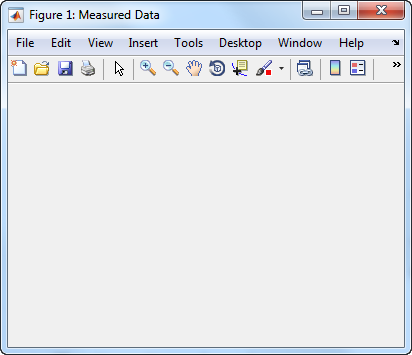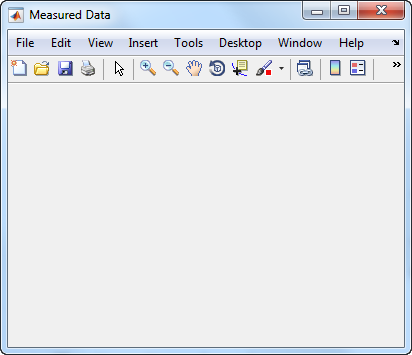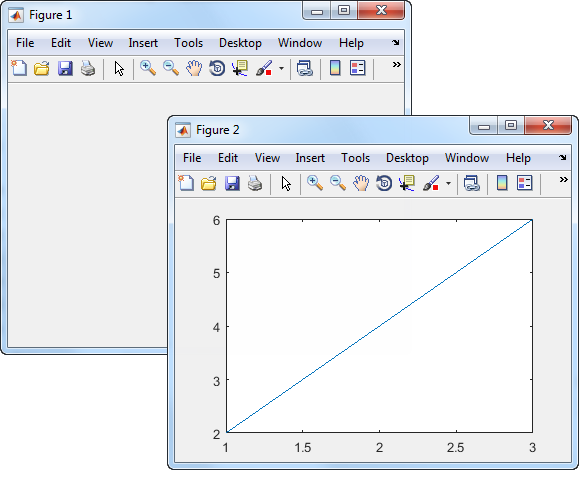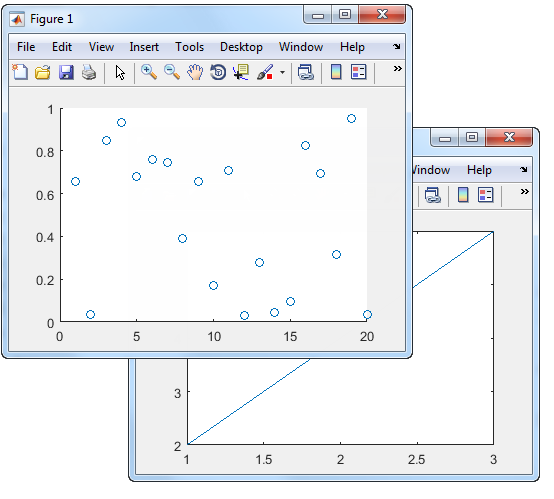• Python 多figure显示与循环中显示figure的问题
2020-12-09 22:49:53

利用 matplotlib.pyplot 进行画图，首先要开 figure，我们必须了解是开多少个figure，是不是一起显示，或者一个figure上画几条线，各是什么颜色什么设置的问题。

import matplotlib.pyplot as plt

import numpy as np

# 定义数据

x = np.linspace(-3, 3, 50)

y1 = 2*x + 1

y2 = x**2

# 第一个figure

plt.figure() #先开figure

plt.plot(x, y1)

# 第二个figure

plt.figure(num=3, figsize=(8, 5),) # 尺寸(8,5)

# 第二个figure包含两个函数的显示 直接写在一起即可

plt.plot(x, y2)

plt.plot(x, y1, color='red', linewidth=1.0, linestyle='--') # 虚线

# 显示图像

plt.show()

figure与循环

这里面有个问题，就是每次循环后可以先产生 figure，然后显示 pylab.show()， 这样是每一次循环后画一个 figure，但是在程序执行中必须先关掉 figure，才能接着运行后面的程序。

如果想全部循环完，再所有的图都一起显示，则需要先每次循环产生一个figure，但是不先pylab.show()，即先用循环产生所有的figure，但是先不show 出来。再在所有循环所有figure， 都产生之后，再统一show出来，这样可以提高运行的效率。

第一段code:

我写的用3层隐藏层的全连接神经网络拟合的sinx代码最后一部分，循环训练20000次，每10000次跳出，将训练的sinx与标准的sinx画在一张图上进行比较，可以看到最后 pylab.show() 在每次跳出后，这样在循环的时候每跳出一次就会显示一张图，但是显示的时候程序不会往下跑，每次都需要关掉才能继续跑，比较麻烦。

for i in range(20000):

train_x,train_y=get_train_data()

sess.run(train_op, feed_dict={x:train_x,y:train_y})

if i % 10000 == 0:

times=int(i/10000)

test_x_array=np.arange(0,2*np.pi,0.01)

test_y_array=np.zeros([len(test_x_array)])

ind = 0

for test_x in test_x_array:

test_y=sess.run(net_out, feed_dict={x:test_x,y:1})

np.put(test_y_array,ind,test_y)

ind += 1

plt.figure(times+1)

draw_correct_line()

pylab.plot(test_x_array, test_y_array, '--', label= str(times)+ 'times')

pylab.show()

第二段code：

将最后的 pylab.show() 放到最后，先循环产生多个 figure，最后所有的 figure一起显示，效率较高。

for i in range(20000):

train_x,train_y=get_train_data()

sess.run(train_op, feed_dict={x:train_x,y:train_y})

if i % 10000 == 0:

times=int(i/10000)

test_x_array=np.arange(0,2*np.pi,0.01)

test_y_array=np.zeros([len(test_x_array)])

ind = 0

for test_x in test_x_array:

test_y=sess.run(net_out, feed_dict={x:test_x,y:1})

np.put(test_y_array,ind,test_y)

ind += 1

plt.figure(times+1)

draw_correct_line()

pylab.plot(test_x_array, test_y_array, '--', label= str(times)+ 'times')

pylab.show()更多相关内容
• 完美解决<Figure size 432x288 with 0 Axes>matplotlib
• 1，完整的C#代码 2，MATLAB可运行代码.m文件 3，MATLAB代码编译成dll的文件 4，原始处理图片一张c# MATLAB
• 今天小编就为大家分享一篇解决在pycharm中显示额外的 figure 窗口问题，具有很好的参考价值，希望对大家有所帮助。一起跟随小编过来看看吧
• 融合图像质量的评价指标:图像清晰度 使用方法 fd=num2str(figure_definition(融合图像));
• 从matlab数据图像文件.fig中导出曲线，图像数据：图像文件可以包含多个子图(subplot，subfigure)；输入为图像文件名称，输出分为两级，第一级为子图，第二级为子图中的曲线数据。
• 让Matlab的Figure窗口最大化Figure窗口全屏-maximize.zip Matlab好像没有直接可以使用的函数。 给的这个函数，是通过调用windows的dll实现，非常简单： >> plot >> maximize >> 复制代码注明：本程序有Alain ...matlab
• Matlab如何在figure画图legend中加入分数latex代码？.docx
• EXPORT_FIGURE是一个简单易用的程序，它提供以下功能，以帮助人们根据期刊的标准导出/保存数字。 EXPORT_FIGURE不会生成图形。 它以适合科学出版的方式更改了生成图形的样式属性。 EXPORT_FIGURE： 1）可以在...matlab
• matlab的figure另存为eps变成黑白图片的解决方法.docx
• 1. Python的figure参数主要有： def figure(num=None, # autoincrement if None, else integer from 1-N figsize=None, # defaults to rc figure.figsize dpi=None, # defaults to rc figure.dpi facecolor=None...
• 读取本地文件夹一张彩色图片，保存一张灰度图和一张figure图 到当前文件夹，注意读取的图片要和程序放在同一个文件夹matlab
• Dreamweaver网页怎么插入Figure？Dreamweaver设计网页的时候，想要插入Figure，该怎么插入呢？下面我们就来看看详细的教程，需要的朋友可以参考下
• figure配套使用，用于标签定义figure元素的标题。 用figure和figcaption标签的好处 搜索引擎搜索到figure的时候会知道，这里存放的是图片、图表、照片、代码等媒体，不不会认为这里存放的是文章等其他东西。搜索...
• 噪声测试Noise Figure Measurement Accuracy – The Y-Factor Method
• 在 GUI 中将 MATLAB 2D 图保存到 Excel 的简单应用程序。 GUI 是在 App Designer 中设计的。matlab
• 详细介绍了matlab设置figure的标题的具体方法
• 本代码主要利用MATLAB工具实现MATLAB——设置figure、axes和surface函数属性，简单明了，易于理解
• 该函数可对matlab绘制的figure图进行取出x，y值，以便对原图形进行编辑MATLAB C#
• this technical file is the Noise figure measurement for RF System
• VS2005中在mfc对话框中嵌入一个matlab的figure图形，并且图形可以随窗口的大小的改变而改变 VS2005中在mfc对话框中嵌入一个matlab的figure图形，并且图形可以随窗口的大小的改变而改变
• matlab开发-从MatlabFigure创建Word文档。使用一个matlab图形文件，将图形粘贴到Word文档中，然后…数学
• Figure Eight 2018 年数据科学家报告
• 与 subplot() 类似，position_figure() 将屏幕划分为用于放置图形的矩形窗格。 句法： 位置图（no_rows，no_columns，fig_no） 在哪里'no_rows' 是图形行的总数'no_columns' 是图列的总数'fig_no' 是当前图形的...matlab
• ## figure函数用法

千次阅读 2021-01-06 08:52:39
figure创建图窗窗口 语法 说明 示例 指定图窗标题 同时使用多个图窗 输入参数 名称-值对组参数 详细信息 当前图窗 Python中用法 figure创建图窗窗口 语法 figure figure(Name,Value) f =figure(___)...

目录

语法

说明

示例

指定图窗标题

同时使用多个图窗

Python中用法

figure创建图窗窗口

## 语法

figure

figure(Name,Value)

f = figure(___)

figure(f)

figure(n)

## 说明

figure 使用默认属性值创建一个新的图窗窗口。生成的图窗为当前窗图

figure(Name,Value) 使用一个或多个名称-值对组参数修改图窗的属性。例如，figure('Color','white') 将背景色设置为白色。

f = figure(___) 返回 Figure 对象。可使用 f 在创建图窗后查询或修改其属性。

figure(f) 将f指定的图窗作为当前图窗，并将其显示在其他所有图窗的上面。

figure(n) 查找 Number 属性等于n的图窗，并将其作为当前图窗。如果不存在具有该属性值的图窗，MATLAB® 将创建一个新图窗并将其Number属性设置为 n

## 示例

### 指定图窗标题

创建一个图窗并指定 Name 属性。默认情况下，生成的标题包含图窗编号。

figure('Name','Measured Data');再次指定 Name 属性，但这次将 NumberTitle 属性设置为 'off'。生成的标题不包含图窗编号。

figure('Name','Measured Data','NumberTitle','off');### 同时使用多个图窗

创建两个图窗，然后创建一个线图。默认情况下，plot 命令的目标为当前图窗。

f1 = figure;
f2 = figure;
plot([1 2 3],[2 4 6]);将当前图窗设置为 f1，使其成为下一个绘图的目标。然后创建一个散点图。

figure(f1);
scatter((1:20),rand(1,20));颜色名称短名称RGB 三元组十六进制颜色代码外观
'red''r'[1 0 0]'#FF0000''green''g'[0 1 0]'#00FF00''blue''b'[0 0 1]'#0000FF''cyan''c'[0 1 1]'#00FFFF''magenta''m'[1 0 1]'#FF00FF''yellow''y'[1 1 0]'#FFFF00''black''k'[0 0 0]'#000000''white''w'[1 1 1]'#FFFFFF''none'不适用不适用不适用无颜色

以下是 MATLAB 在许多类型的绘图中使用的默认颜色的 RGB 三元组和十六进制颜色代码。

RGB 三元组十六进制颜色代码外观
[0 0.4470 0.7410]'#0072BD'[0.8500 0.3250 0.0980]'#D95319'[0.9290 0.6940 0.1250]'#EDB120'[0.4940 0.1840 0.5560]'#7E2F8E'[0.4660 0.6740 0.1880]'#77AC30'[0.3010 0.7450 0.9330]'#4DBEEE'[0.6350 0.0780 0.1840]'#A2142F'可绘制区域的位置和大小，指定为 [left bottom width height] 形式的向量。此区域不包括图窗边框、标题栏、菜单栏和工具栏。此表介绍 Position 向量中的每个元素。

元素说明
left从主画面左边缘到窗口的内部左边缘的距离。在具有多个监视器的系统上，此值可能为负数。

如果图窗已停靠，则此值相对于 MATLAB 桌面中的“图窗”面板。
bottom从主画面下边缘到窗口的内部下边缘的距离。在具有多个监视器的系统上，此值可能为负数。

如果图窗已停靠，则此值相对于 MATLAB 桌面中的“图窗”面板。
widthfigure 的左右内部边缘之间的距离。
height窗口的上下内部边缘之间的距离。

所有测量值都采用Units属性指定的单位。图窗停靠时不能指定图窗的Position属性。

在 MATLAB Online™ 中，Position向量的bottomleft元素被忽略。要放置包括边框、标题栏、菜单栏和工具栏在内的整个窗口，请使用OuterPosition属性。

注意

Windows® 操作系统会强制应用最小窗口宽度和最大窗口大小。如果指定的图窗尺寸超出这些限制，将按照这些限制而不是指定的尺寸显示图窗。

单位值说明
'pixels'（默认值）

像素。

从 R2015b 开始，以像素为单位的距离不再依赖 Windows 和 Macintosh 系统上的系统分辨率：

• 在 Windows 系统上，一个像素是 1/96 英寸。

• 在 Macintosh 系统上，一个像素是 1/72 英寸。

在 Linux® 系统上，一个像素的大小由系统分辨率确定。

'normalized'这些单位依据父容器进行归一化。容器的左下角映射到 (0,0)，右上角映射到 (1,1)
'inches'英寸。
'centimeters'厘米。
'points'磅。1 磅等于 1/72 英寸。
'characters'

这些单位基于图形根对象的默认 uicontrol 字体：

• 字符宽度 = 字母 x 的宽度。

• 字符高度 = 两个文本行的基线之间的距离。

要访问默认的 uicontrol 字体，请使用 get(groot,'defaultuicontrolFontName') 或 set(groot,'defaultuicontrolFontName')

MATLAB 从父对象的左下角测量所有单位。此属性会影响Position属性。如果更改 Units 属性，请考虑在完成计算后将其值还原为默认值，以免影响采用默认值的其他函数。

指定UnitsPosition属性的顺序具有以下影响：

• 如果在 Position属性之前指定 Units，则 MATLAB 会使用指定的单位来设置 Position

• 如果在 Position 属性之后指定 Units 属性，则 MATLAB 会使用默认的Units来设置 position。然后，MATLAB 将 Position 值转换为指定的单位表示的等价值。

## Python中用法

figure(num=None, figsize=None, dpi=None, facecolor=None, edgecolor=None, frameon=True)
• num:图像编号或名称，数字为编号 ，字符串为名称
• figsize:指定figure的宽和高，单位为英寸；
• dpi参数指定绘图对象的分辨率，即每英寸多少个像素，缺省值为80 。1英寸等于2.5cm,A4纸是 21*30cm的纸张 。
• facecolor：背景颜色
• edgecolor：边框yanse
• frameon：是否显示边框

例如：

import matplotlib.pyplot as plt
# 创建自定义图像
fig=plt.figure(figsize=(4,3),facecolor='blue')
plt.show()

展开全文matlab 矩阵 开发语言...